# How to Find Isosceles Triangle Angles

Angles in an Isosceles Triangle• An isosceles triangle is a type of triangle that has two sides that are the same length.
• The two marked sides are both the same length.
• The two angles opposite these two marked sides are also the same: both angles are 70°.
• All three interior angles add to 180° because it is a triangle.

An isosceles triangle has two equal sides and angles.

These two equal sides will be marked with short lines.• The triangle above is isosceles because there are lines marking two of its equal sides.
• Angle ‘a’ and the angle marked 50° are opposite the two equal sides.
• Angle ‘a’ must be 50° as well.
• The two equal angles, 50° and 50°, add to make 100°.
• To find angle ‘b’, we subtract 100° from 180°. This equals 80°.# Angles in an Isosceles Triangle

## What is an Isosceles Triangle?

An isosceles triangle is a triangle that has two equal sides and two equal angles. The two equal sides are marked with lines and the two equal angles are opposite these sides.

We can recognise an isosceles triangle because it will have two sides marked with lines.

Below is an example of an isosceles triangle.

It has two equal sides marked with a small blue line.

It has two equal angles marked in red.We can see that in this above isosceles triangle, the two base angles are the same size.

All isosceles triangles have a line of symmetry in between their two equal sides.

The sides that are the same length are each marked with a short line.

The two equal angles are opposite to the two equal sides.

The angle at which these two marked sides meet is the odd one out and therefore is different to the other two angles.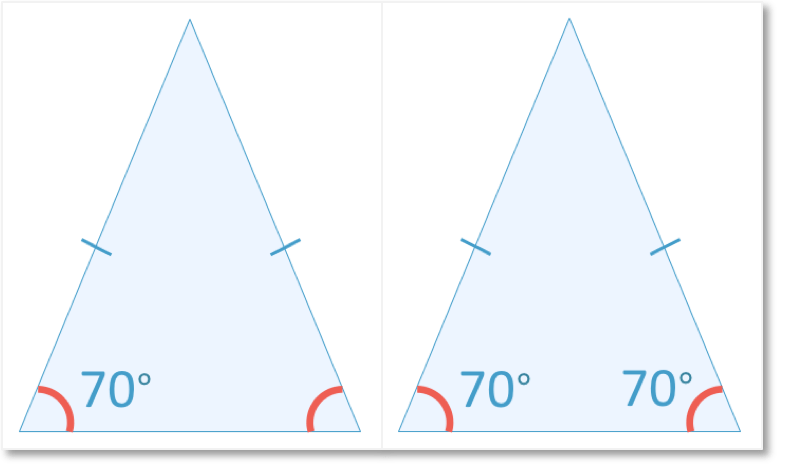If we are told that one of these marked angles is 70°, then the other marked angle must also be 70°.

## How to Find a Missing Angle in an Isosceles Triangle

To find a missing angle in an isosceles triangle use the following steps:

• If the missing angle is opposite a marked side, then the missing angle is the same as the angle that is opposite the other marked side.
• If the missing angle is not opposite a marked side, then add the two angles opposite the marked sides together and subtract this result from 180.

This is because all three angles in an isosceles triangle must add to 180°

For example, in the isosceles triangle below, we need to find the missing angle at the top of the triangle.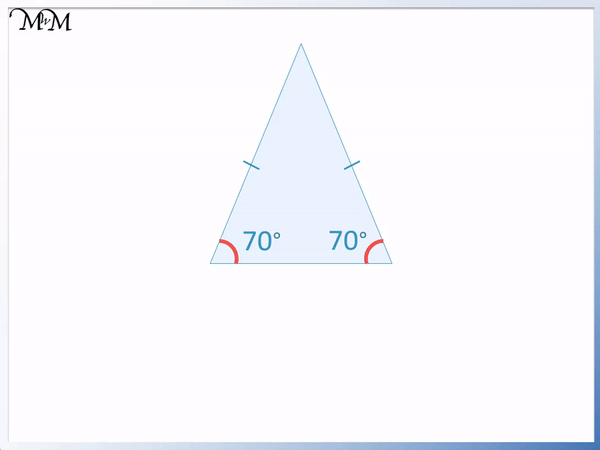The two base angles are opposite the marked lines and so, they are equal to each other.

Both base angles are 70 degrees.

The missing angle is not opposite the two marked sides and so, we add the two base angles together and then subtract this result from 180 to get our answer.

70° + 70° = 140°

The two base angles add to make 140°.

Angles in an isosceles triangle add to 180°.

We subtract the 140° from 180° to see what the size of the remaining angle is.

180° – 140° = 40°The missing angle on the top of this isosceles triangle is 40°.

We can also think, “What angle do we need to add to 70° and 70° to make 180°?”

## How to Find Missing Angles in an Isosceles Triangle from only One Angle

If only one angle is known in an isosceles triangle, then we can find the other two missing angles using the following steps:

• If the known angle is opposite a marked side, then the angle opposite the other marked side is the same. Add these two angles together and subtract the answer from 180° to find the remaining third angle.
• If the known angle is not opposite a marked side, then subtract this angle from 180° and divide the result by two to get the size of both missing angles.

Here is an example of finding two missing angles in an isosceles triangle from just one known angle.

We know that one angle is 50°. This angle is opposite one of the marked sides.

This means that it is the same size as the angle that is opposite the other marked side. This is angle ‘a’.

Therefore angle ‘a’ is 50° too.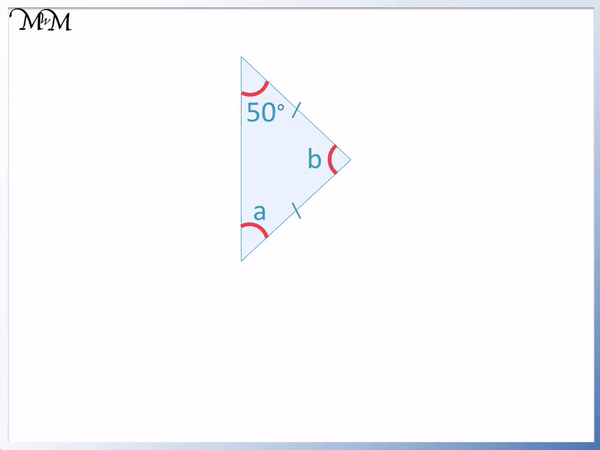Now to find angle ‘b’, we use the fact that all three angles add up to 180°.

To find angle ‘b’, we subtract both 50° angles from 180°. We first add the two 50° angles together.

50° + 50° = 100°

and 180° – 100° = 80°

Angle ‘b’ is 80° because all angles in a triangle add up to 180°.

Here is another example of finding the missing angles in isosceles triangles when one angle is known.

This time, we know the angle that is not opposite a marked side. We have 30°.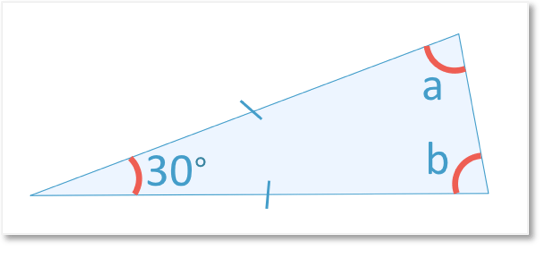We can subtract 30° from 180° to see what angle ‘a’ and ‘b’ add up to.

180° – 30° = 150°

And so, angles ‘a’ and ‘b’ both add up to 150°.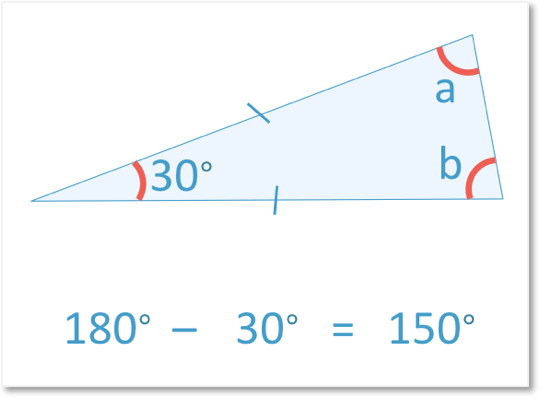Because angles ‘a’ and ‘b’ are both opposite the marked sides, they are equal to each other.

The size of these two angles are the same.

We divide 150° into two equal parts to see what angle ‘a’ and ‘b’ are equal to.

150° ÷ 2 = 75°

This is because 75° + 75° = 150°.Angles ‘a’ and ‘b’ are both 75°.We can see that the three angles in an isosceles triangle add up to 180°.

75° + 75° + 30 = 180°.Now try our lesson on Forming Algebraic Expressions where we learn how to write algebraic expressions.